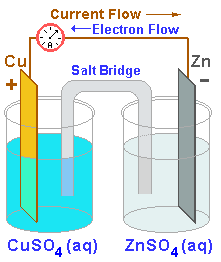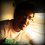# Physical Chemistry - Electrochemistry - 1INTRODUCTION
The connection between chemistry and electricity is a very old one, going back to Allesandro Volta's discovery in 1793, that electricity could be produced by placing two dissimilar metals on opposite sides of a moistened paper.

Oxidation-Reduction reactions involve a transfer of electrons. Since a flow of electrons constitutes an electric current, we can, at least in principle, use any redox reaction to produce an electric current.

Conversely, we can use an electric current to carry out redox reactions that do not proceed spontaneously.

ELECTRODE POTENTIAL
If you were to place a zinc rod into a solution containing zinc ions, an equilibrium would be established between them.
There is a tendency for zinc atoms on the surface of the rod to be attracted into the solution. However, they don't enter the solution as atoms, but as zinc ions ($Zn^{+2}$). In this guise, they can be solvated by water molecules. The electrons left behind when a zinc atom is transformed into a positive ion, remain on the rod.

As a result, the region very close to the rod suffers an increase in positive charge, while the rod carries a layer of negative charge. Whenever there is a separation of positive and negative charges, we should be able to measure a Potential Difference. All we need is a voltmeter.

However, it is not as easy as it seems. This idea has a flaw in it. As soon as we dip the terminals of the voltmeter into the solution, another equilibrium is set up. This time, it is between the metal of the terminals and the ions it gives in the solution. This phenomenon disturbs the readings of the voltmeter dramatically.

Thus, the best we can do is, to agree to keep one of such setups as constant, and always measure the difference between this one and the others. The system that has been chosen as a standard is the Standard Hydrogen Electrode. We shall refer to this as the S.H.E.

In the second post, we shall discuss the S.H.E in a bit more detail, and also learn how to calculate Standard Electrode Potential (i.e Potenial w.r.t S.H.E)

Read the Next Post here : Physical Chemistry - Electrochemistry - 2Note by Anish Puthuraya
7 years, 5 months ago

This discussion board is a place to discuss our Daily Challenges and the math and science related to those challenges. Explanations are more than just a solution — they should explain the steps and thinking strategies that you used to obtain the solution. Comments should further the discussion of math and science.

When posting on Brilliant:

• Use the emojis to react to an explanation, whether you're congratulating a job well done , or just really confused .
• Ask specific questions about the challenge or the steps in somebody's explanation. Well-posed questions can add a lot to the discussion, but posting "I don't understand!" doesn't help anyone.
• Try to contribute something new to the discussion, whether it is an extension, generalization or other idea related to the challenge.

MarkdownAppears as
*italics* or _italics_ italics
**bold** or __bold__ bold
- bulleted- list
• bulleted
• list
1. numbered2. list
1. numbered
2. list
Note: you must add a full line of space before and after lists for them to show up correctly
paragraph 1paragraph 2

paragraph 1

paragraph 2

[example link](https://brilliant.org)example link
> This is a quote
This is a quote
    # I indented these lines
# 4 spaces, and now they show
# up as a code block.

print "hello world"
# I indented these lines
# 4 spaces, and now they show
# up as a code block.

print "hello world"
MathAppears as
Remember to wrap math in $$ ... $$ or $ ... $ to ensure proper formatting.
2 \times 3 $2 \times 3$
2^{34} $2^{34}$
a_{i-1} $a_{i-1}$
\frac{2}{3} $\frac{2}{3}$
\sqrt{2} $\sqrt{2}$
\sum_{i=1}^3 $\sum_{i=1}^3$
\sin \theta $\sin \theta$
\boxed{123} $\boxed{123}$

Sort by:

Wow, looking forward to your next post.

- 7 years, 5 months ago

It is good, I never used to understand what electrode potential really is!!!

- 7 years, 5 months ago

Superb post, Anish.

- 7 years, 5 months ago

Awesome post!

- 7 years, 5 months ago